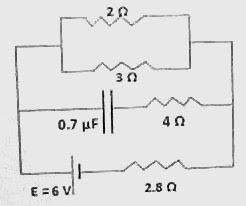## Sunday, March 30, 2008

### Two Karnataka CET Multiple Choice Questions on Direct Current Circuits

Questions on direct current circuits have been discussed on this site on various occasions. You can find all those posts by clicking on the label ‘direct current circuit’ below this post. The search box at the top of this page can also be used to access topics of your choice.

The following multiple choice questions appeared in Karnataka CET 2004 question paper:

(1) In the circuit shown, the internal resistance of the cell is negligible. The steady state current in the 2 Ω resistor is(a) 0.6 A

(b) 1.2 A

(c) 0.9 A

(d) 1.5 A

In steady state, the capacitor is fully charged and no current flows into its branch. So, you can ignore the branch containing the capacitor and the 4 Ω resistor. The circuit thus reduces to the 6 V cell in series with the 2.8 Ω resistor and the parallel combined value of 2 Ω and 3 Ω [ which is 2×3/(2+3) = (6/5) Ω = 1.2 Ω].

The current sent by the cell is 6 V/ (2.8 + 1.2) Ω = (6/4) A = 1.5 A.

This current gets divided between the 2Ω and 3Ω resistor paths. The current (I) through the 2Ω resistor is given by

I = (Main current × Resistance of the other branch)/ Total resistance

[Remember the above relation for the branch current when current gets divided between two branches].

Therefore, we have

I = (1.5×3)/(2+3) = 0.9 A.

(2) An unknown resistance R1 is connected in series with a resistance of 10 Ω. This combination is connected to one gap of a metre bridge while a resistance R2 is connected in the other gap. The balance point is at 50 cm. Now, when the 10 Ω resistor is removed and R1 alone is connected, the balance point shifts to 40 cm. The value of R1 is (in ohms)

(a) 20

(b) 10

(c) 60

(d) 40

The condition for the balance of a metre bridge (which is basically a Wheatstone bridge) is

P/Q = L/(100 – L) where P and Q are the resistances in the two gaps and the balancing length L cm is measured from the side of P.

In the above problem we have

(R1 + 10) /R2 = 1 since the balance point is at the middle of the wire when one gap contains the series combination of R1 and 10 Ω and the other gap contains R2.

With R1 alone in the gap (on removing the 10 Ω resistance), the balance condition is

R1/R2 = 40/60

From the above two equations (on dividing one by the other), we have

(R1 + 10) /R1 = 60/40, from which R1 = 20 Ω.